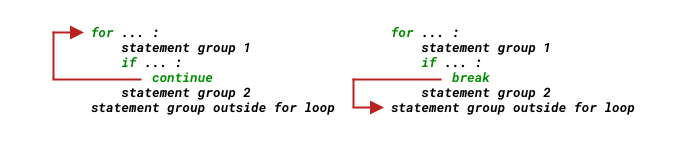Article Outline

流程控制

for n in range(2, 100):
if n == 2:
print(n)
continue
for i in range(2, n):
if (n % i) == 0:
break
else:
print(n)

if 语句

if 语句的最简单构成是这样 —— 注意第 1 行末尾的冒号 : 和第 2 行的缩进：

if expression:
statements

import random
r = random.randrange(1, 1000)

if r % 2 == 0:
print(f'{r} is even.')
372 is even.

if expression:
statements_for_True
else:
statements_for_False

import random
r = random.randrange(1, 1000)

if r % 2 == 0:
print(f'{r} is even.')
else:
print(f'{r} is odd.')
945 is odd.

if expression_1:
statements_for_expression_1_True

elif expression_2:
statements_for_expression_2_True

elif expression_3:
statements_for_expression_3_True

elif expression_...:
statements_for_expression_..._True

Python 用 elif 处理这种多情况分支，相当于其它编程语言中使用 switch 或者 case……

elifelse if 的缩写，作用相同。

import random
r = random.randrange(2, 13)

if r == 7:
print('Draw!')
elif r < 7:
print('Small!')
elif r > 7:
print('Big!')
Big!

import random
r = random.randrange(0, 13) # 生成的随机数应该从 0 开始了；

if r == 7:
print('Draw!')
elif r >= 2 and r < 7:      # 如果这里直接写 elif r < 7:，那么，else: 那一部分永远不会被执行……
print('Small!')
elif r > 7:
print('Big!')
else:
print('Not valid!')
Small!

for 循环

Python 语言中，for 循环不使用其它语言中那样的计数器，取而代之的是 range() 这个我称其为 “整数等差数列生成器” 的函数。

for( a = 0; a < 10; a = a + 1 ){
printf("value of a: %d\n", a);
}

for a in range(10):
print(f'value of a: {a}') #每次 a 的值都不同，从 0 递增至 9
value of a: 0
value of a: 1
value of a: 2
value of a: 3
value of a: 4
value of a: 5
value of a: 6
value of a: 7
value of a: 8
value of a: 9

range() 函数

range() 是个内建函数，它的文档是这样写的：

range(stop)

range(start, stop[, step])

print(range(10))
print(list(range(10))) # 将 range(10) 转换成 list，以便清楚看到其内容。
range(0, 10)
[0, 1, 2, 3, 4, 5, 6, 7, 8, 9]

start 参数的默认值是 0。如需指定起点，那么得给 range() 传递两个参数，比如，range(2, 13)……

print(list(range(2, 13)))
[2, 3, 4, 5, 6, 7, 8, 9, 10, 11, 12]

for i in range(1, 10, 2):
print(i)
1
3
5
7
9

a = list(range(0, -10, -1))
print(a)
[0, -1, -2, -3, -4, -5, -6, -7, -8, -9]

Continue、Break 和 Pass

for n in range(2, 100):
if n == 2:
print(n)
continue
for i in range(2, n):
if (n % i) == 0:
break
else:
print(n)

continue 语句将忽略其后的语句开始下次循环，而 break 语句将从此结束当前循环，开始执行循环之后的语句：for 语句块还可以附加一个 else —— 这是 Python 的一个比较有个性的地方。附加在 for 结尾的 else 语句块，_在没有 break 发生的情况下会运行_。

for n in range(2, 100):
if n == 2:
print(n)
continue
for i in range(2, n):
if (n % i) == 0:
break
else:               # 下一行的 print(n) 事实上属于语句块 for i in range(2, n):
print(n)        # 整个循环结束，都没有发生 break 的情况下，才执行一次 print(n)
2
3
5
7
11
13
17
19
23
29
31
37
41
43
47
53
59
61
67
71
73
79
83
89
97

for n in range(2, 100):
if n == 2:
print(n)
continue
for i in range(2, n):
if (n % i) == 0:
break
print(n)            # 事实上相当于针对 range(2, 100) 中每个 n 都执行了一次 print(n)
# 这个 print(n) 属于语句块 for n in range(2, 100):
for n in range(2, 100):
if n == 2:
print(n)
continue
for i in range(2, n):
if (n % i) == 0:
break
print(n)            # 事实上相当于针对 range(2, n) 中每个 i 都执行了一次 print(n)
2
3
5
5
5
7
7
7
7
7
9
11
11
11
...
97
97
97
97
99

pass 语句什么都不干：

def someFunction():
pass

for i in range(100):
pass
if i % 2 == 0:
pass

while 循环

• Collection-controlled loops（以集合为基础的循环）
• Condition-controlled loops（以条件为基础的循环）

while 循环的格式如下：

while expression:
statements

n = 1000
a, b = 0, 1
while a < n:
print(a, end=' ')
a, b = b, a+b
print()
0 1 1 2 3 5 8 13 21 34 55 89 144 233 377 610 987

forwhile 的区别在哪里？什么时候应该用哪个？

for 更适合处理序列类型的数据（Sequence Type）的迭代，比如处理字符串中的每一个字符，比如把 range() 返回的数列当作某种序列类型的索引。

while 更为灵活，因为它后面只需要接上一个逻辑表达式即可。

一个投骰子赌大小的游戏

• 每次计算机随机生成一个 2... 12 之间的整数，用来模拟机器人投两个骰子的情况；
• 机器人和用户的起始资金都是 10 个硬币
• 要求用户猜大小：
• 用户输入 b 代表 “大”；
• 用户输入 s 代表 “小”；
• 用户输入 q 代表 “退出”；
• 用户的输入和随机产生的数字比较有以下几种情况：
• 随机数小于 7，用户猜小，用户赢；
• 随机数小于 7，用户猜大，用户输；
• 随机数等于 7，用户无论猜大还是猜小，结局平，不输不赢；
• 随机数大于 7，用户猜小，用户输；
• 随机数大于 7，用户猜大，用户赢；
• 游戏结束条件：
• 机器人和用户，若任意一方硬币数量为 0，则游戏结束；
• 用户输入了 q 主动终止游戏。
from random import randrange

coin_user, coin_bot = 10, 10 # 可以用一个赋值符号分别为多个变量赋值
rounds_of_game = 0

def bet(dice, wager):    # 接收两个参数，一个是骰子点数，另一个用户的输入
if dice == 7:
print(f'The dice is {dice};\nDRAW!\n') # \n 是换行符号
return 0
elif dice < 7:
if wager == 's':
print(f'The dice is {dice};\nYou WIN!\n')
return 1
else:
print(f'The dice is {dice};\nYou LOST!\n')
return -1
elif dice > 7:
if wager == 's':
print(f'The dice is {dice};\nYou LOST!\n')
return -1
else:
print(f'The dice is {dice};\nYou WIN!\n')
return 1

while True:         #  除 for 之外的另外一个循环语句
print(f'You: {coin_user}\t Bot: {coin_bot}')
dice = randrange(2, 13)   # 生成一个 2 到 12 的随机数
wager = input("What's your bet? (type 'q' to quit)")
if wager == 'q':
break
elif wager in 'bs':  # 只有当用户输入的是 b 或者 s 得时候，才 “掷骰子”……
result = bet(dice, wager)
coin_user += result    # coin_user += result 相当于 coin_user = coin_user + result
coin_bot -= result
rounds_of_game += 1
if coin_user == 0:
print("Woops, you've LOST ALL, and game over!")
break
elif coin_bot == 0:
print("Woops, the robot's LOST ALL, and game over!")
break

print(f"You've played {rounds_of_game} rounds.\n")
print(f"You have {coin_user} coins now.\nBye!")

总结

• 只处理一种情况，用 if ...
• 处理 True/False 两种情况，用 if ... else ...
• 处理多种情况，用 if ... elif ... elif ... else ...
• 迭代有序数据类型，用 for ... in ...，如果需要处理没有 break 发生的情况，用 for ... else ...
• 其它循环，用 while ...
• 与循环相关的语句还有 continuebreakpass
• 函数从控制流角度去看其实就是子程序